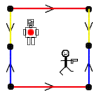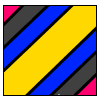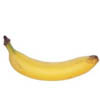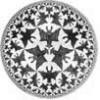# Resources tagged with: Non Euclidean Geometry

Filter by: Content type:
Age range:
Challenge level:

There are 10 NRICH Mathematical resources connected to Non Euclidean Geometry, you may find related items under 3D Geometry, Shape and Space.

Broad Topics > 3D Geometry, Shape and Space > Non Euclidean Geometry### Weird Universes

##### Age 16 to 18Challenge Level

Consider these weird universes and ways in which the stick man can shoot the robot in the back.### Torus Patterns

##### Age 16 to 18Challenge Level

How many different colours would be needed to colour these different patterns on a torus?### Curvature of Surfaces

##### Age 16 to 18

How do we measure curvature? Find out about curvature on soccer and rugby balls and on surfaces of negative curvature like banana skins.### Over the Pole

##### Age 16 to 18Challenge Level

Two places are diametrically opposite each other on the same line of latitude. Compare the distances between them travelling along the line of latitude and travelling over the nearest pole.### Spherical Triangles on Very Big Spheres

##### Age 16 to 18Challenge Level

Shows that Pythagoras for Spherical Triangles reduces to Pythagoras's Theorem in the plane when the triangles are small relative to the radius of the sphere.### Pythagoras on a Sphere

##### Age 16 to 18Challenge Level

Prove Pythagoras' Theorem for right-angled spherical triangles.### Flight Path

##### Age 16 to 18Challenge Level

Use simple trigonometry to calculate the distance along the flight path from London to Sydney.### When the Angles of a Triangle Don't Add up to 180 Degrees

##### Age 14 to 18

This article outlines the underlying axioms of spherical geometry giving a simple proof that the sum of the angles of a triangle on the surface of a unit sphere is equal to pi plus the area of the. . . .### How Many Geometries Are There?

##### Age 16 to 18

An account of how axioms underpin geometry and how by changing one axiom we get an entirely different geometry.### Geometry and Gravity 1

##### Age 11 to 18

This article (the first of two) contains ideas for investigations. Space-time, the curvature of space and topology are introduced with some fascinating problems to explore.